# Quantitative Aptitude Quiz for SSC (Mix): 19 April 2021

Updated Mon, 19 Apr 2021 07:10 PM ISTSource: safalta
1. A total of 518 candidates appeared in an examination. 78% of the boys and 92% of the girls passed the examination. If the total pass percentage was 83%, find the number of boys who appeared in the examination.

### Free Demo Classes

a) 315
b)405
c) 333
d) 388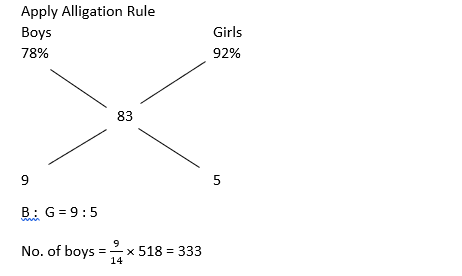2. A' buys 54 kg of sugar at the rate of Rs. 11.50 per kg and 76 kg of sugar at the rate of Rs. 13.5 per kg. He mixes the both type of sugar. At what price (per kg) should he sell the mixture to get a profit of 30% on the cost price?

a) 16.47
b) 15.5
c) 18.53
d) 15.67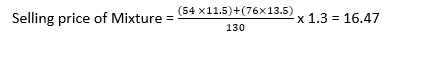3. If 1000 copies of a book of 13 sheets require 26 reams of paper, how much reams of paper is required for 5000 copies of a book of 17 sheets?
a) 510
b) 170
c) 340
d) 1404. The average annual salary of Assistants and Engineers in a factory is Rs. 12500, whereas the average annual salary of all the 6 Engineers is Rs. 24500. If the average annual salary of the Assistants is Rs. 9500, then find the number of Assistants
a) 12
b) 18
c) 24
d) 30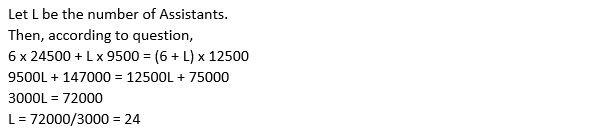5. A deceptive vintner mixes 30 litres of water with 120 litres of alcohol. After selling one-third of this mixture, he adds water to replenish the quantity that he has sold. What is the current proportion of water to alcohol?
a) 8 : 7
b) 4 : 5
c) 5 : 4
d) 7 : 8

150 litres of mixture has 120 litres of alcohol and 30 litres of water.
When 50 litres of mixture is taken out, it contains 40 litres of alcohol and 10 litres of water.
So, alcohol left = 80 litres and water left = 20 litres.
If 50 litres of water is added, then ratio of water to alcohol = 70 : 80 = 7 : 8

6. One alloy of brass is composed of tin, copper and zinc in the ratio 5 : 7 : 11, respectively. Another alloy of brass contains these metals in the ratio 21 : 18 : 7. Equal weights of these alloys are taken and fused together. What is the ratio of these ingredient metals in the resulting alloy?

a) 27:35:18
b) 26:25:15
c) 31:32:29
d) 52:50:36

Let alloy A be the first alloy.
So, 23 kg of alloy A has 5 kg of tin, 7 kg of copper and 11 kg of zinc.
92 kg of alloy A has 20 kg of tin, 28 kg of copper and 44 kg of zinc.
Similarly, 92 kg of the second alloy (alloy B) has 42 kg of tin, 36 kg of copper and 14 kg of zinc
On fusing alloys A and B together, we get 184 kg of alloy C, which has (20 + 42 = 62) kg of tin, (28 + 36 = 64) kg of copper and (44 + 14 = 58 ) kg of zinc.
Thus, the ratio of the ingredient metals in the resulting alloy is 62:64:58 = 31:32:29

7. Three containers A, B and C have mixture of milk and water in the ratios of 2 : 7, 5 : 7 , and 5 : 13, respectively. The capacities of the containers are in the ratio of 5 : 6 : 7. If the contents of all the three containers are mixed together, then find the ratio of milk to water in the resulting mixture

a) 25 : 56
b)28 : 53
c)24 : 77
d) 48 : 113

Let the volume of container A be 180v, that of B be 216v, and that of C be 252v.
Thus, total volume of fluid when the contents are mixed together = 180v + 216v + 252v = 648v
So, amount of milk in container A = 40v
Amount of milk in container B = 90v
Amount of milk in container C = 70v
Total amount of milk = 200v
Thus, total amount of water = 648v - 200v = 448v
Thus, required ratio = 200 : 448 = 25 : 56

8. One alloy of metal contains 75% copper and 25% tin. Another alloy contains 69% copper and 13% tin. Later, they are mixed, so that the mixture contains 23% of tin. What percent of copper will it contain?

a) 82%
b) 98 %
c) 92%
d) 74%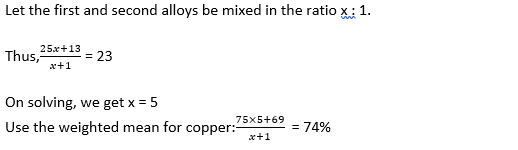9. There are two qualities of gold: 19carats and 22 carats, having different prices per gram, their weights being 125 g and 175 g, respectively. After equal amounts of gold were taken from both, the gold removed from 19carats was added to the 22 carat gold and vice versa. Now, the resulting two types of gold have the same price per gram. Find the amount of gold drawn out from each type of gold.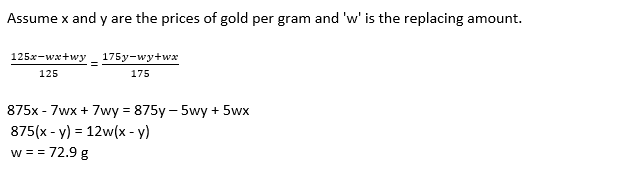a) 72.9g
b) 75.68g
c) 78.62
d) 82.6g

10. Kanhaiya gets some coins made of an alloy of gold and silver. The alloy with a weight of 300 gm contains 27% of gold. What weight of another gold-silver alloy containing 37% of silver must be alloyed with the first piece of alloy in order to obtain a new alloy with 48% of gold?

a) 150g
b) 148g
c) 175g
d) 185g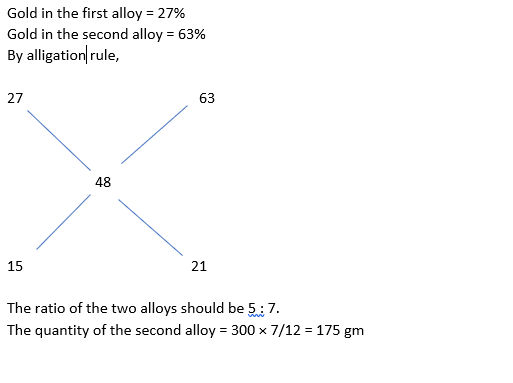### Disclaimer

अपनी वेबसाइट पर हम डाटा संग्रह टूल्स, जैसे की कुकीज के माध्यम से आपकी जानकारी एकत्र करते हैं ताकि आपको बेहतर अनुभव प्रदान कर सकें, वेबसाइट के ट्रैफिक का विश्लेषण कर सकें, कॉन्टेंट व्यक्तिगत तरीके से पेश कर सकें और हमारे पार्टनर्स, जैसे की Google, और सोशल मीडिया साइट्स, जैसे की Facebook, के साथ लक्षित विज्ञापन पेश करने के लिए उपयोग कर सकें। साथ ही, अगर आप साइन-अप करते हैं, तो हम आपका ईमेल पता, फोन नंबर और अन्य विवरण पूरी तरह सुरक्षित तरीके से स्टोर करते हैं। आप कुकीज नीति पृष्ठ से अपनी कुकीज हटा सकते है और रजिस्टर्ड यूजर अपने प्रोफाइल पेज से अपना व्यक्तिगत डाटा हटा या एक्सपोर्ट कर सकते हैं। हमारी Cookies Policy, Privacy Policy और Terms & Conditions के बारे में पढ़ें और अपनी सहमति देने के लिए Agree पर क्लिक करें।

Agree

Get free counselling session